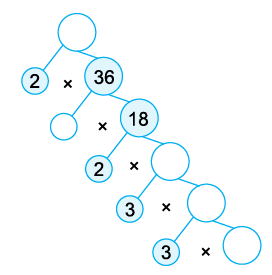# Worksheet: HCF & LCM Notes | Study Mathematics for Class 5 - Class 5

## Class 5: Worksheet: HCF & LCM Notes | Study Mathematics for Class 5 - Class 5

The document Worksheet: HCF & LCM Notes | Study Mathematics for Class 5 - Class 5 is a part of the Class 5 Course Mathematics for Class 5.
All you need of Class 5 at this link: Class 5

Question 1: Answer the following questions:

• Write the first 3 multiples of 21.
• Which of the following numbers are composite?
2, 7, 15, 27, 29, 37, 53, 69, 87.

Question 2: Write two prime numbers whose difference is 1.

Question 3: Write all composite numbers between 90 and 100.

Question 4: Which of the following pairs are coprime?

• 18 and 30
• 35 and 52

Question 5: Is it possible that a number is divisible by 8 but not divisible by 4?

Question 6: Which of these numbers are divisible by 3 or 4 or both?

• 87,342
• 23,148

Question 7: Test the divisibility of the following numbers by 3:

• 90,82,746
• 70,335

Question 8: Test the divisibility of the following numbers by 5:

• 2,01,234
• 4,37,839

Question 9: Test the divisibility of the following numbers by 9:

• 3,478
• 8,74,512

Question 10: Test the divisibility of the following numbers by 11:

• 1,00,01,001
• 5,335

Question 11: In each of the following numbers, replace * by the smallest number to make it divisible by 9:

• 66784 *
• 5321 * 43

Question 12: Test the divisibility of the following numbers by 4:

• 7,314
• 9,31,105

Question 13: Find the H.C.F. of:

• 14 and 35
• 15 and 35
• 30, 75 and 90
• 8 and 40

Question 14: Find all the common factors of:

• 16 and 40
• 18 and 45
• 12 and 15
• 52 and 117

Question 15: Find the L.C.M. of the following numbers:

• 5 and 8
• 4 and 9
• 12 and 15
• 26 and 15
• 8, 10 and 12
• 6 and 10
• 9 and 11

Question 16: Write in the product form:

• 24 × 52 × 32
• 65 × 26 × 32
• 67 × 105
• 48

Question 17: Fill the vacant circles in each of the following factor trees.Question 18: Find the HCF of the following numbers by long division method:

• 40, 75
• 24, 64
• 27, 45
• 81, 117
• 18, 45, 36
• 54, 96, 120
• 70, 98, 154

Question 19: Find the L.C.M. by prime factorisation method:

• 15 and 20
• 24 and 36
• 42 and 91
• 50 and 105
• 4, 36 and 52
• 10, 35 and 40
• 12 and 18

Question 20: Answer the following questions:

• The HCF of two numbers is 19 and their LCM is 228. If one of the numbers is 57, find the other.
• The product of two numbers is 25,024 and their H.C.F. is 8. Find their L.C.M.
The document Worksheet: HCF & LCM Notes | Study Mathematics for Class 5 - Class 5 is a part of the Class 5 Course Mathematics for Class 5.
All you need of Class 5 at this link: Class 5Use Code STAYHOME200 and get INR 200 additional OFF

## Mathematics for Class 5

10 videos|54 docs|35 tests

Track your progress, build streaks, highlight & save important lessons and more!

,

,

,

,

,

,

,

,

,

,

,

,

,

,

,

,

,

,

,

,

,

;